×#### Thank you for registering.

One of our academic counsellors will contact you within 1 working day.

Click to Chat

1800-1023-196

+91-120-4616500

CART 0

• 0

MY CART (5)

Use Coupon: CART20 and get 20% off on all online Study Material

ITEM
DETAILS
MRP
DISCOUNT
FINAL PRICE
Total Price: Rs.

There are no items in this cart.
Continue Shopping• Complete JEE Main/Advanced Course and Test Series
• OFFERED PRICE: Rs. 15,900
• View Details

```Chapter 10: Sine and Cosine Formulae and Their Applications – Exercise 10.1

Sine and Cosine Formulae and Their Applications – Exercise 10.1 – Q.1

∠A = 45°,∠B = 60° and ∠C = 75°

Using sine rule,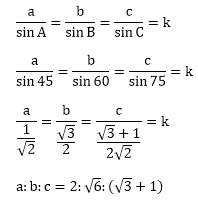Sine and Cosine Formulae and Their Applications – Exercise 10.1 – Q.2

∠C = 105°, ∠B = 45°, a = 2

From here we can calculate that

∠A = 30°

a sin B = b sin A

⟹ 2sin 45 = b sin 30Sine and Cosine Formulae and Their Applications – Exercise 10.1 – Q.3

a = 18, b = 24, c = 30, ∠C = 90°Sine and Cosine Formulae and Their Applications – Exercise 10.1 – Q.4Let a = k sin A, b = k sin B (Using sine rule)

LHSSine and Cosine Formulae and Their Applications – Exercise 10.1 – Q.5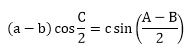Let a = k sin A, B = k sin B, c = k sin C

LHS,Sine and Cosine Formulae and Their Applications – Exercise 10.1 – Q.6LHS,= RHS

Sine and Cosine Formulae and Their Applications – Exercise 10.1 – Q.7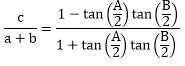LHS,= RHS

Sine and Cosine Formulae and Their Applications – Exercise 10.1 – Q.8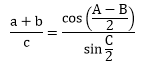Let a = k sin A, b = k sin B, c = k sin C

LHS,Sine and Cosine Formulae and Their Applications – Exercise 10.1 – Q.9Let a = k sin A, b = k sin B, c = k sin C

RHS,Sine and Cosine Formulae and Their Applications – Exercise 10.1 – Q.10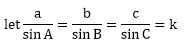LHS,```### Course Features

• 728 Video Lectures
• Revision Notes
• Previous Year Papers
• Mind Map
• Study Planner
• NCERT Solutions
• Discussion Forum
• Test paper with Video Solution